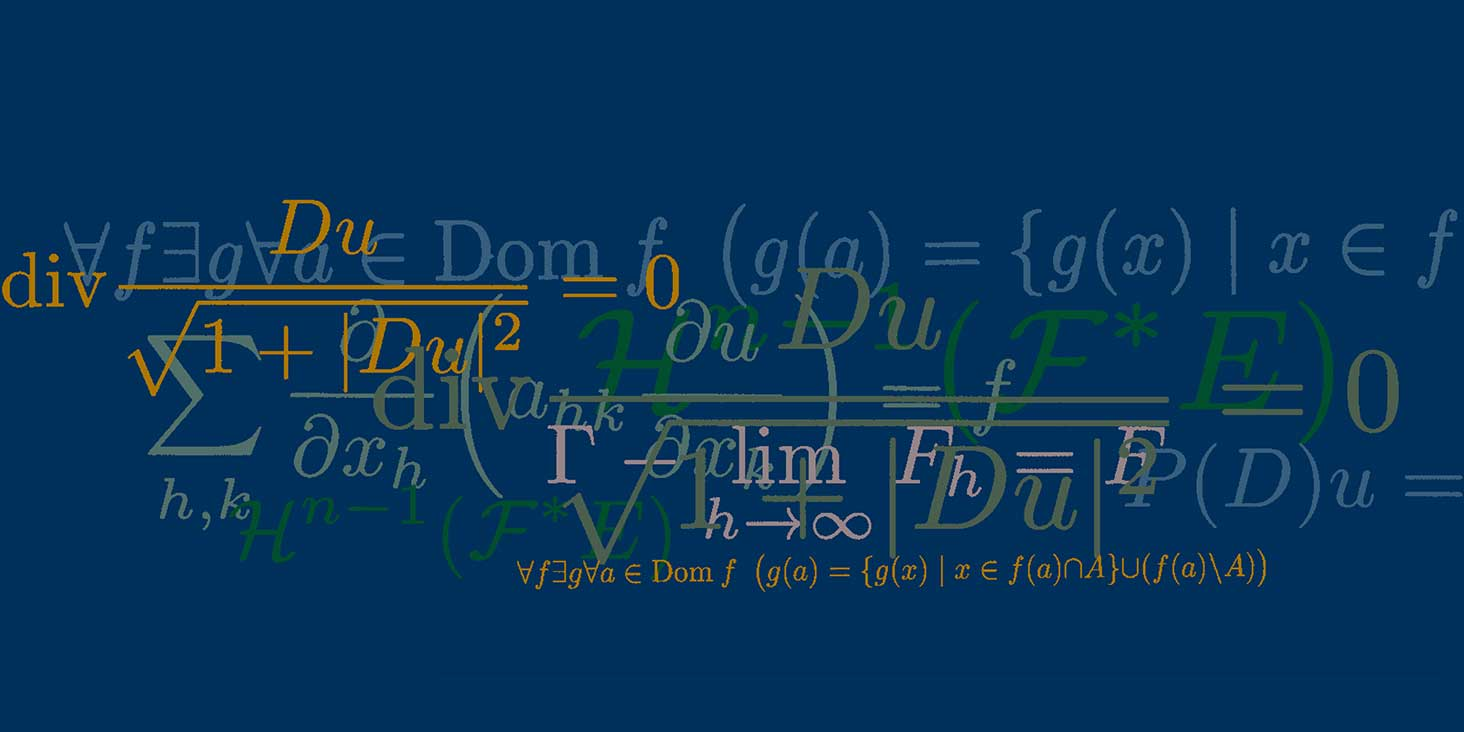# Numerical solutions methods for problems of PDE-constrained optimisation

Colloquio De Giorgi

## Speakers

Andrew Wathen
University of Oxford

## Contatti

Andrew Wathen - University of Oxford
Numerical solutions methods for problems of PDE-constrained optimisation

Abstract
Since the advent of computers there has been interest in numerical methods for solving partial differential equations (PDE) problems---both methods of approximation and numerical linear algebra for the resulting sets of equations which are usually of very large dimension. Sometimes it is the solutions themselves that are of interest, but there is a significant class of problems where there is a design optimization criterion with physical constraints that are expressed in terms of PDEs; it is always the motion of fluids described by flow equations which provide the principal constraints when an engineer designs an aerodynamic structure with minimal drag and acceptable lift for example. In this talk I will consider numerical methods for such optimization problems with PDE constraints, in particular methods of iterative numerical linear algebra that render feasible the solution of such problems on advanced computers.

The event will take place in person.
Please note that, for organizational purposes, registration is mandatory.